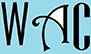## Free Online Math Videos

This Webpage provides math videos meant to assist disadvantaged students in special education programs & special education with special needs and college students who need help with math strategies.  These are solving signs, PEMDAS, slope intercept form, order of operation, fraction, & solve for X questions that they can work on or refer to in order to do their math homework or answer math problems on their math worksheets.

These videos below answer problems that deal with solving math signs, PEMDAS, slope intercept form, order of operation, fraction, & solve for X questions when you click on each link.  Here are a list of the most popular and most common solving math signs, PEMDAS, slope intercept form, order of operation, fraction, & solve for X problems that can be found in many math homework and math exams.  The answers for the solving signs, PEMDAS, slope intercept form, order of operation, fraction, & solve for X questions are explained in steps and the answers are easily understood.